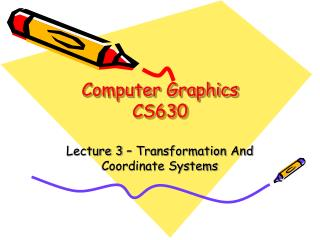DownloadDownload PresentationComputer Graphics CS630

# Computer Graphics CS630

Download Presentation## Computer Graphics CS630

- - - - - - - - - - - - - - - - - - - - - - - - - - - E N D - - - - - - - - - - - - - - - - - - - - - - - - - - -
##### Presentation Transcript

1. Computer GraphicsCS630 Lecture 3 – Transformation And Coordinate Systems

2. What is a Transformation? • Maps points (x, y) in one coordinate system to points (x', y') in another coordinate system x' = ax + by + c y' = dx + ey + f

3. Transformations • Simple transformation • Translation • Rotation • Scaling

4. Transformations • Deformable transformations • Shearing • Tapering • Twisting • Etc.. • Issues • Can be combined • Are these operations invertible?

5. Transformations • Why use transformations? • Position objects in a scene (modeling) • Change the shape of objects • Create multiple copies of objects • Projection for virtual cameras • Animations

6. Classes of Transformation • Rigid-Body/ Euclidean transformation • Similarity Transforms • Linear Transforms • Affine Transforms • Projective Transforms

7. Rigid-Body / Euclidean Transforms • Preserves distances • Preserves angles Rigid / Euclidean Identity Translation Rotation

8. How are Transforms Represented? x' = ax + by + c y' = dx + ey + f x y c f x' y' a b d e + = p' = M p + t

9. Translation = + = +

10. Properties of Translation = = = =

11. Scaling Uniform scaling iff

12. Rotations (2D)

13. Rotations 2D • So in matrix notation

14. Rotations (3D)

15. Properties of Rotations order matters!

16. Combining Translation & Rotation

17. Combining Translation & Rotation

18. Homogeneous Coordinates • Add an extra dimension • in 2D, we use 3 x 3 matrices • In 3D, we use 4 x 4 matrices • Each point has an extra value, w a e i m b f j n c g k o d h l p x y z w x' y' z' w' = p' = M p

19. Homogeneous Coordinates • Most of the time w = 1, and we can ignore it a e i 0 b f j 0 c g k 0 d h l 1 x y z 1 x' y' z' 1 =

20. Homogeneous Coordinates can be represented as where

21. Translation Revisited

22. Rotation & Scaling Revisited

23. Transforming Tangents

24. Transforming Normals

25. Surface Normal • Surface Normal: unit vector that is locally perpendicular to the surface

26. Why is the Normal important? • It's used for shading — makes things look 3D! Diffuse Shading object color only

27. ± x = Red± y = Green± z = Blue Visualization of Surface Normal

28. How do we transform normals? nWS nOS World Space Object Space

29. Transform the Normal like the Ray? • translation? • rotation? • isotropic scale? • scale? • reflection? • shear? • perspective?

30. More Normal Visualizations Incorrect Normal Transformation Correct Normal Transformation

31. Transforming Normals

32. Rotations about an arbitrary axis Rotate by around a unit axis

33. An Alternative View • We can view the rotation around an arbitrary axis as a set of simpler steps • We know how to rotate and translate around the world coordinate system • Can we use this knowledge to perform the rotation?

34. Rotation about an arbitrary axis • Translate the space so that the origin of the unit vector is on the world origin • Rotate such that the extremity of the vector now lies in the xz plane (x-axis rotation) • Rotate such that the point lies in the z-axis (y-axis rotation) • Perform the rotation around the z-axis • Undo the previous transformations

35. Rotation about an arbitrary axis • Step 1 Rotate x-axis y (a,b,c) x x (a’,b’,c’) z

36. Closer Look at Y-Z Plane • Need to rotate  degrees around the x-axis y  z

37. Equations for 

38. Rotation about the Y-axis • Using the same analysis as before, we need to rotate  degrees around the Y-axis y x (a’,b’,c’)=Rx () (a,b,c)T z

39. Equations for 

40. Rotation about the Z-axis • Now, it is aligned with the Z-axis, thus we can simply rotate  degrees around the Z-axis. • Then undo all the transformations we just did

41. Equation summary

42. Deformations Transformations that do not preserve shape • Non-uniform scaling • Shearing • Tapering • Twisting • Bending

43. Shearing

44. Tapering

45. Twisting

46. Bending

47. Quick Recap • Computer Graphics is using a computer to generate an image from a representation. computer Model Image

48. Modeling • What we have been studying so far is the mathematics behind the creation and manipulation of the 3D representation of the object. computer Model Image

49. What have we seen so far? • Basic representations (point, vector) • Basic operations on points and vectors (dot product, cross products, etc.) • Transformation – manipulative operators on the basic representation (translate, rotate, deformations) – 4x4 matrices to “encode” all these.